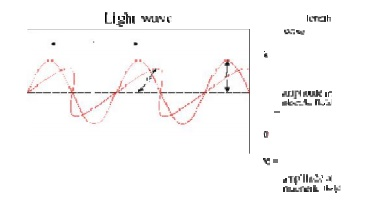Home | | Remote Sensing Techniques and GIS | Important Questions and Answers - EMR And Its Interaction With Atmophere And Earth Material

# Important Questions and Answers - EMR And Its Interaction With Atmophere And Earth Material

Civil - Remote Sensing Techniques and GIS - EMR and Its Interaction With Atmosphere and Earth Material

EMR And Its Interaction With Atmophere And Earth  Material

1.What is Remote Sensing?

Remote sensing is  the science and art of obtaining information about object,  area,  or  phenomena through the analysis of data acquired by a device that is not  in contact with the  object, area, or phenomena under investigation.

2.What are all the applications of remote sensing?

In many  respects,  remote  sensing  can be thought of as a  reading process. Using various sensors, we remotely collect data that may be analyzed to obtain information  about the  objects,  areas,or  phenomena beinginvestigated.

The remotely collected data can be of  many forms,   including Variations in    force distributions, acoustic wave distributions, or electromagnetic energy distributions.

3.Write the physics  of remote sensing ?

Visible light is only one   of many  forms of Electromagnetic energy. Radio waves, heat, ultraviolet rays, and X-rays are other   familiar forms. All this energy is inherently similar and radiates in accordance with basic wave theory. This theory describes electromagnetic energy    as traveling  in  harmonic,  sinusoidal  fashion  at  the  'velocity  of  light'   c.  The distance from one  wave peak to  the next is the  wave  length ?,  and the number  of peaks passing  a fixed point in  space   per   unit time  is the wave frequency V.

From  basic physics, wave obey the general equation

C = v y

4.What are the Components of Remote Sensing ?Electromagnetic  (EM)  radiation  is  a  self-propagating  wave in space or through  matter.  EM  radiation  has  an  electric and magnetic field  component which  oscillate in phase perpendicular to  each  other and to the direction of energy propagation.

6.Write the type of Electromagnetic radiation?

Electromagnetic radiation is  classified into types according to the frequency  of the  wave, these types include (in  order of increasing frequency): radio     waves,  microwaves,   terahertz radiation, infrared radiation,  visible light, ultraviolet  radiation,  X-rays and gamma  rays.

7.Draw the quantum theory interaction?

A quantum theory  of  the interaction  between electromagnetic radiation and   matter   such   as   electrons   is   described   by the theory of   quantum electrodynamics.In   refraction,      a  wave       crossing      from   one   medium       to      another       of different density   alters its speed     and    direction upon      entering the new  medium.

The  ratio of  the  refractive   indices          of      the   media  determines  the     degree         of refraction, and is summarized by Snell's law. Light disperses into a visible spectrum as light is shone through a prism because of refraction.

9.Draw the Wave model?10.Write Planck's equation?

The    frequency    of  the  wave  is   proportional          to      the     magnitude   of the particle's          energy.        Moreover,   because  photons  are    emitted        and absorbed by charged          particles, they  act as transporters of energy.

The energy  per  photon can be calculated  by Planck's  equation:

where E is the energy, h is Planck's constant, and f is frequency.

11.What is Black body ?

By definition a black body is a material that absorbs all the radiant energy that strikes it. A black body also radiates the maximum amount of energy, which is dependent on the kinetic temperature.

12.Write Stefan Boltzman law?

According to the Stefan-Boltzman law the radiant flux of a black body, Fb, at a kinetic temperature, Tkin, is Fb = s* Tkin 4 where s is the Stefan- Boltzman constant, 5.67*10-12 W*cm-2* o K-4.

13.What is emissivity?

Emissivity is a measure of the ability of a material to both radiate and absorb energy. Materials with a high emissivity absorb and radiate large proportions of incident and kinetic energy, respectively (and vice-versa).

14.Write Wein's Displacement law?

For an object at a constant temperature the radiant power peak refers to the wavelength at which the maximum amount of energy is radiated, which is expressed as lmax. The sun, with a surface temperature of almost 6000 o K, has its peak at 0.48mm (wavelength of yellow). The average surface temperature of the earth is 290 o K (17 o C), which is also called the ambient temperature; the peak concentration of energy emitted from the earth is at 9.7mm.This shift to longer wavelengths with decreasing temperature is described by Wien's displacement law, which states:

lmax = 2,897mm o K /Trad o K

15.Write Planck's Law?

The primary law governing blackbody radiation is the Planck Radiation Law, which governs the intensity of radiation emitted by unit surface area into a fixed direction (solid angle) from the blackbody as a function of wavelength for a fixed temperature. The Planck Law can be expressed through the following equation.

16.What is  Scattering?

Scattering occurs when particles or large gas molecules present in the atmosphere interact with and cause the electromagnetic radiation to be redirected from its original path. How much scattering takes place depends on several factors including the wavelength of the radiation, the abundance of particles or gases, and the distance the radiation travels through the atmosphere. There are three (3) types of scattering which take place.

17.What are the types of scattering?

(i) Rayleigh scattering occurs when particles are very small compared to the wavelength of the radiation.

(ii) Mie scattering It occurs when the particles are just about the same size as the wavelength of the radiation.

(iii) Non Selective Scattering

The final scattering mechanism of importance is called nonselective scattering. This occurs when the particles are much larger than the wavelength of the radiation.

18.What is Atmospheric Windows?

The areas of the spectrum which are not severely influenced by atmospheric absorption and thus, are useful to remote sensors, are called atmospheric windows.

Study Material, Lecturing Notes, Assignment, Reference, Wiki description explanation, brief detail
Civil : Remote Sensing Techniques and GIS : EMR and Its Interaction With Atmosphere and Earth Material : Important Questions and Answers - EMR And Its Interaction With Atmophere And Earth Material |